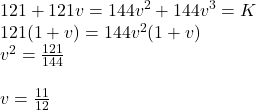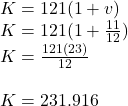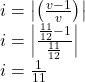## At an effective annual interest rate i, i > 0, each of the following two sets of payments has present value K: a. A payment

Question

At an effective annual interest rate i, i > 0, each of the following two sets of payments has present value K:

a. A payment of 121 immediately and another payment of 121 at the end of one year
b. A payment of 144 at the end of two years and another payment of 144 at the end of three vears.

Calculate i.

in progress 0
2 weeks 2021-08-30T03:46:53+00:00 1 Answers 0 views 0

Hence the calculated

Step-by-step explanation:

Now,Then K is calculated as,Here we get,